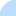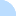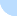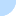## DebcheckDebcheck - bullseye  Summary  All packages (light) main only  Broken PreDepends  Broken Depends  Broken Recommends  Broken Suggests  HalfBroken PreDepends  HalfBroken Depends  HalfBroken Recommends  HalfBroken Suggests  Build-Depends  HalfBroken Build-Depends  Malformed Build-Depends  OldLibs  Priority  Old Standards-Version  No Standards-Version  Wrong Standards-Version Syntax other  Depends Main on !Main all sections  Broken PreDepends  Broken Depends  Broken Recommends  Broken Suggests  HalfBroken PreDepends  HalfBroken Depends  HalfBroken Recommends  HalfBroken Suggests  Build-Depends  HalfBroken Build-Depends  Malformed Build-Depends  OldLibs  Priority  Old Standards-Version  No Standards-Version  Wrong Standards-Version SyntaxDistribution: sid bookworm bullseye buster stretch jessie

# Problems for source package mono-debugger-libs

Current Source Version: 0+20131201.3459502-1.1
Current Maintainer: Jo Shields <directhex@apebox.org>
Section: main
BTS entry: https://bugs.debian.org/src:mono-debugger-libs

# Binary Package: libmono-debugging-soft-cil (Version: 0+20131201.3459502-1.1)

BTS entry: https://bugs.debian.org/libmono-debugging-soft-cil

### Half Broken Relationships

(not necessarily bugs)

Package has a Depends on libc6.1 (>= 2.31) which cannot be satisfied on ppc64el. At least one of libc6 (>= 2.31) | libc6.1 (>= 2.31) | libc0.1 (>= 2.31) can be satisfied however.
Package has a Depends on libc6.1 (>= 2.31) which cannot be satisfied on s390x. At least one of libc6 (>= 2.31) | libc6.1 (>= 2.31) | libc0.1 (>= 2.31) can be satisfied however.
Package has a Depends on libc6.1 (>= 2.31) which cannot be satisfied on arm64. At least one of libc6 (>= 2.31) | libc6.1 (>= 2.31) | libc0.1 (>= 2.31) can be satisfied however.
Package has a Depends on libc6.1 (>= 2.31) which cannot be satisfied on mips64el. At least one of libc6 (>= 2.31) | libc6.1 (>= 2.31) | libc0.1 (>= 2.31) can be satisfied however.
Package has a Depends on libc6.1 (>= 2.31) which cannot be satisfied on mipsel. At least one of libc6 (>= 2.31) | libc6.1 (>= 2.31) | libc0.1 (>= 2.31) can be satisfied however.
Package has a Depends on libc6.1 (>= 2.31) which cannot be satisfied on armel. At least one of libc6 (>= 2.31) | libc6.1 (>= 2.31) | libc0.1 (>= 2.31) can be satisfied however.
Package has a Depends on libc6.1 (>= 2.31) which cannot be satisfied on i386. At least one of libc6 (>= 2.31) | libc6.1 (>= 2.31) | libc0.1 (>= 2.31) can be satisfied however.
Package has a Depends on libc6.1 (>= 2.31) which cannot be satisfied on armhf. At least one of libc6 (>= 2.31) | libc6.1 (>= 2.31) | libc0.1 (>= 2.31) can be satisfied however.
Package has a Depends on libc6.1 (>= 2.31) which cannot be satisfied on amd64. At least one of libc6 (>= 2.31) | libc6.1 (>= 2.31) | libc0.1 (>= 2.31) can be satisfied however.
Package has a Depends on libc0.1 (>= 2.31) which cannot be satisfied on amd64. At least one of libc6 (>= 2.31) | libc6.1 (>= 2.31) | libc0.1 (>= 2.31) can be satisfied however.
Package has a Depends on libc0.1 (>= 2.31) which cannot be satisfied on armel. At least one of libc6 (>= 2.31) | libc6.1 (>= 2.31) | libc0.1 (>= 2.31) can be satisfied however.
Package has a Depends on libc0.1 (>= 2.31) which cannot be satisfied on i386. At least one of libc6 (>= 2.31) | libc6.1 (>= 2.31) | libc0.1 (>= 2.31) can be satisfied however.
Package has a Depends on libc0.1 (>= 2.31) which cannot be satisfied on armhf. At least one of libc6 (>= 2.31) | libc6.1 (>= 2.31) | libc0.1 (>= 2.31) can be satisfied however.
Package has a Depends on libc0.1 (>= 2.31) which cannot be satisfied on mipsel. At least one of libc6 (>= 2.31) | libc6.1 (>= 2.31) | libc0.1 (>= 2.31) can be satisfied however.
Package has a Depends on libc0.1 (>= 2.31) which cannot be satisfied on s390x. At least one of libc6 (>= 2.31) | libc6.1 (>= 2.31) | libc0.1 (>= 2.31) can be satisfied however.
Package has a Depends on libc0.1 (>= 2.31) which cannot be satisfied on ppc64el. At least one of libc6 (>= 2.31) | libc6.1 (>= 2.31) | libc0.1 (>= 2.31) can be satisfied however.
Package has a Depends on libc0.1 (>= 2.31) which cannot be satisfied on arm64. At least one of libc6 (>= 2.31) | libc6.1 (>= 2.31) | libc0.1 (>= 2.31) can be satisfied however.
Package has a Depends on libc0.1 (>= 2.31) which cannot be satisfied on mips64el. At least one of libc6 (>= 2.31) | libc6.1 (>= 2.31) | libc0.1 (>= 2.31) can be satisfied however.

# Binary Package: libmono-debugging-cil (Version: 0+20131201.3459502-1.1)

BTS entry: https://bugs.debian.org/libmono-debugging-cil

### Half Broken Relationships

(not necessarily bugs)

Package has a Depends on libc0.1 (>= 2.31) which cannot be satisfied on armhf. At least one of libc6 (>= 2.31) | libc6.1 (>= 2.31) | libc0.1 (>= 2.31) can be satisfied however.
Package has a Depends on libc0.1 (>= 2.31) which cannot be satisfied on armel. At least one of libc6 (>= 2.31) | libc6.1 (>= 2.31) | libc0.1 (>= 2.31) can be satisfied however.
Package has a Depends on libc0.1 (>= 2.31) which cannot be satisfied on i386. At least one of libc6 (>= 2.31) | libc6.1 (>= 2.31) | libc0.1 (>= 2.31) can be satisfied however.
Package has a Depends on libc0.1 (>= 2.31) which cannot be satisfied on amd64. At least one of libc6 (>= 2.31) | libc6.1 (>= 2.31) | libc0.1 (>= 2.31) can be satisfied however.
Package has a Depends on libc0.1 (>= 2.31) which cannot be satisfied on arm64. At least one of libc6 (>= 2.31) | libc6.1 (>= 2.31) | libc0.1 (>= 2.31) can be satisfied however.
Package has a Depends on libc0.1 (>= 2.31) which cannot be satisfied on mips64el. At least one of libc6 (>= 2.31) | libc6.1 (>= 2.31) | libc0.1 (>= 2.31) can be satisfied however.
Package has a Depends on libc0.1 (>= 2.31) which cannot be satisfied on s390x. At least one of libc6 (>= 2.31) | libc6.1 (>= 2.31) | libc0.1 (>= 2.31) can be satisfied however.
Package has a Depends on libc0.1 (>= 2.31) which cannot be satisfied on ppc64el. At least one of libc6 (>= 2.31) | libc6.1 (>= 2.31) | libc0.1 (>= 2.31) can be satisfied however.
Package has a Depends on libc0.1 (>= 2.31) which cannot be satisfied on mipsel. At least one of libc6 (>= 2.31) | libc6.1 (>= 2.31) | libc0.1 (>= 2.31) can be satisfied however.
Package has a Depends on libc6.1 (>= 2.31) which cannot be satisfied on mipsel. At least one of libc6 (>= 2.31) | libc6.1 (>= 2.31) | libc0.1 (>= 2.31) can be satisfied however.
Package has a Depends on libc6.1 (>= 2.31) which cannot be satisfied on arm64. At least one of libc6 (>= 2.31) | libc6.1 (>= 2.31) | libc0.1 (>= 2.31) can be satisfied however.
Package has a Depends on libc6.1 (>= 2.31) which cannot be satisfied on mips64el. At least one of libc6 (>= 2.31) | libc6.1 (>= 2.31) | libc0.1 (>= 2.31) can be satisfied however.
Package has a Depends on libc6.1 (>= 2.31) which cannot be satisfied on s390x. At least one of libc6 (>= 2.31) | libc6.1 (>= 2.31) | libc0.1 (>= 2.31) can be satisfied however.
Package has a Depends on libc6.1 (>= 2.31) which cannot be satisfied on ppc64el. At least one of libc6 (>= 2.31) | libc6.1 (>= 2.31) | libc0.1 (>= 2.31) can be satisfied however.
Package has a Depends on libc6.1 (>= 2.31) which cannot be satisfied on amd64. At least one of libc6 (>= 2.31) | libc6.1 (>= 2.31) | libc0.1 (>= 2.31) can be satisfied however.
Package has a Depends on libc6.1 (>= 2.31) which cannot be satisfied on armhf. At least one of libc6 (>= 2.31) | libc6.1 (>= 2.31) | libc0.1 (>= 2.31) can be satisfied however.
Package has a Depends on libc6.1 (>= 2.31) which cannot be satisfied on armel. At least one of libc6 (>= 2.31) | libc6.1 (>= 2.31) | libc0.1 (>= 2.31) can be satisfied however.
Package has a Depends on libc6.1 (>= 2.31) which cannot be satisfied on i386. At least one of libc6 (>= 2.31) | libc6.1 (>= 2.31) | libc0.1 (>= 2.31) can be satisfied however.

Last updated: Fri Feb 3 15:27:30 2023

DO NOT MASS FILE BUG REPORTS
GET A CONSENSUS ON debian-devel@lists.debian.org BEFORE MASS FILING BUGS